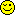## Trivia

Subject:
And one more solution
Response To:

Alex Y
There is another theorem (also unknown to me) that for two intersecting perpendicular chords, the sum of the squares of the four segments is 4*(r^2). So once you have used 3*x=2*6 to find the unstated chord segment to be 4, this theorem says that:
4*(r^2) = 4^2 + 3^2 + 2^2 + 6^2
=16+9+4+36 = 65
So r = sqrt(65)/2

These two theorems that I didn't know about sure make this easier!